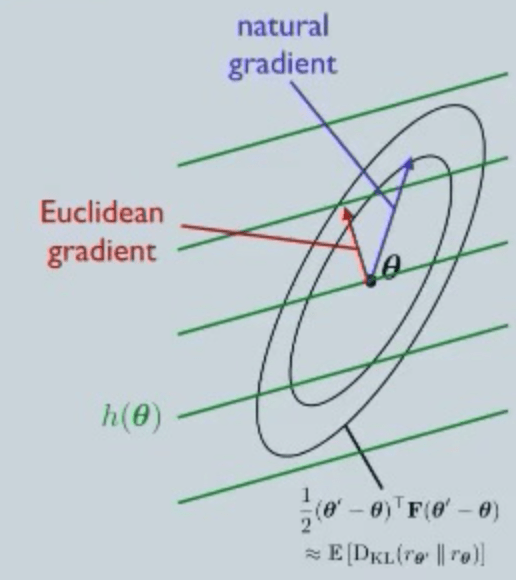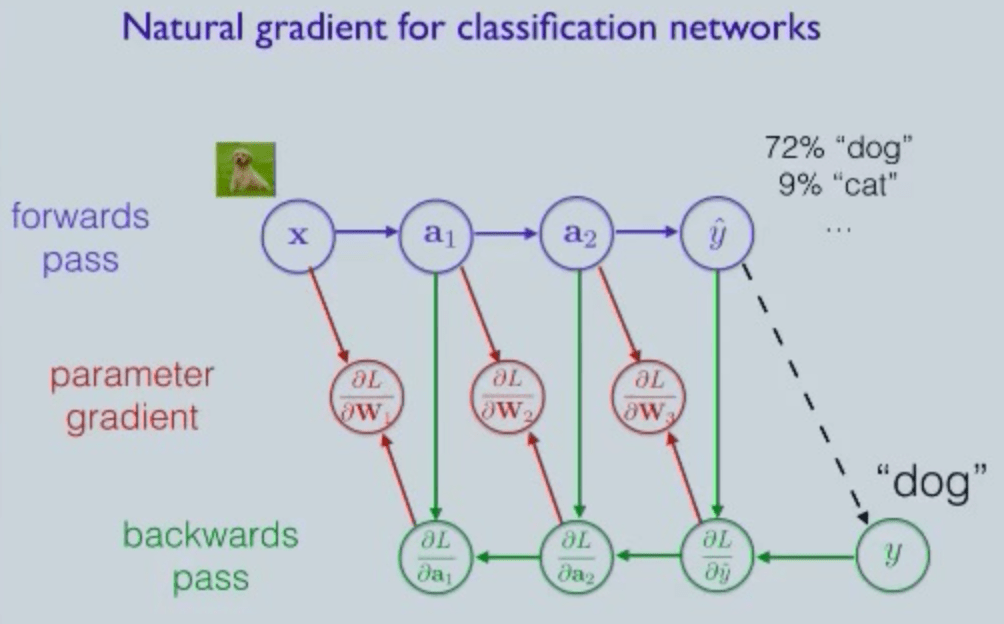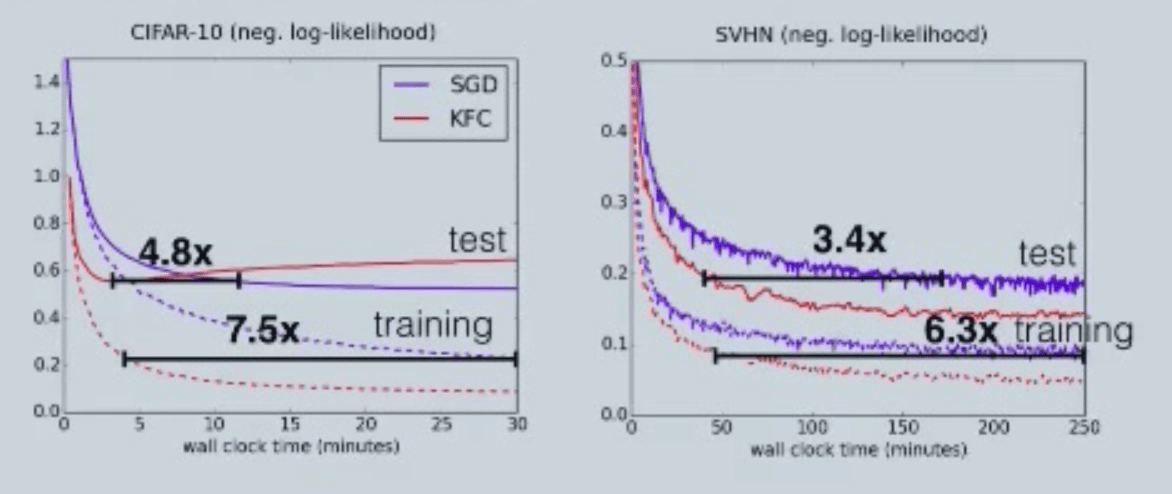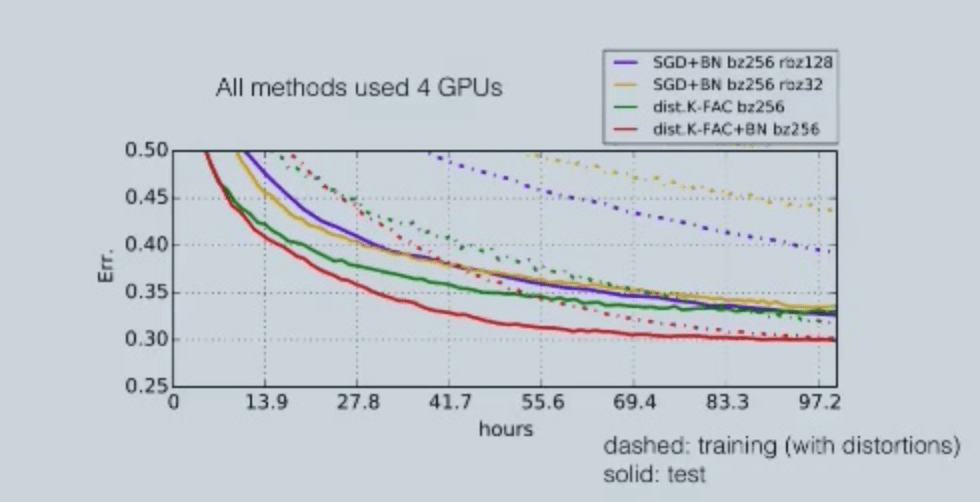# Optimizing Neural Networks using Structured Probabilistic Models

This talk presents natural gradient, a new approach to approximate the Fisher matrix by adopting structured probabilistic models.

## Abstract

Neural networks have recently driven significant progress in a wide range of machine learning applications such as vision, speech, and text understanding. Despite much engineering effort to boost the computational efficiency of neural net training, most networks are still trained using variants of stochastic gradient descent (SGD). This talk presents natural gradient, a second-order training method and a new approach to approximate the Fisher matrix by adopting structured probabilistic models. Using probabilistic modeling assumptions motivated by the structure of the computation graph and empirical analysis of the distribution over derivatives, approximations to the Fisher matrix could allow for efficient approximation of the natural gradient. The resulting optimization algorithm is invariant to some common reparameterizations of neural networks, suggesting that it automatically enjoys the computational benefits of these reparameterizations.

## IntroductionTake map projections as an example, every method of projection produces distortions to some degree. This is because a map is just a representation for one of the reference surfaces used to model the Earth, or the globe. The same reasoning can be applied to neural networks. When we train a neural network, our goal is to learn a function, but we have to parameterize the function in terms of weights and biases. Such processes generate distortions, and current optimizers frequently used in training methods, such as stochastic gradient descent (SGD), cannot not handle them. Those first-order optimizers are known to have slow convergence problems when the curvature is badly conditioned. Since they only use gradient information but not curvature information, they will oscillate in directions with high curvature and make slow progress in directions with low curvature. Natural gradient, on the other hand, measures the gradient on the “globe” and not on the “map”, thus is better at dealing with the distortions.

For two neural networks, the “distance” between them can be measured as the average Kullback–Leibler divergence (a measure of the non-symmetric difference between two probability distributions P and Q) between their predictive distributions. The Kullback–Leibler divergence, again, cannot be measured exactly. Thus, the Fisher matrix is used as the second Taylor approximation of the average KL divergence to depict the distance between two neural networks. Recall from statistics, the Fisher matrix equals the covariance of log-likelihood derivatives with respect to the weights of the network. (For more information, see Amari, 1998)Gradient ascent, also known as the steepest ascent in Euclidean norm, depends on parameterization. That is to say, when we represent the same function with distinct parameterizations, gradient ascent will give completely different results.
Natural gradient ascent, a second-order optimization method, has the potential to speed up training by correcting for the curvature of the loss function. It is the steepest ascent in Fisher norm (as shown by the figure above), thus is invariant to reparameterization. However, despite this advantage, natural gradient is not suitable for large dataset and large neural network model, since the dimension of Fisher matrix depends on the number of trainable parameters. Because modern neural networks can have tens of millions of parameters, they make the size of Fisher matrix extremely large. Thus, we need to find a way to further approximate the natural gradient ascent.

## A new approach: Probabilistic models of the gradient computation

There are several methods to approximate the second-order Taylor training, such as the diagonal method, iterative method, and subspace-based method. Unfortunately, these methods are either too slow in computing speed or too expensive in space and memory. The presenter thus rendered a more structured method that could represent the Fisher matrix more accurately — the probabilistic models of the gradient computation. Since Fisher matrix is the covariance matrix of the log-likelihood gradient, approximation of Fisher matrix will depend on gradients. If we draw samples of the gradient, we could see that the gradient is high in large curvature and small in low curvature. The new approach takes the distribution of gradients into account instead of approximating the values.

Since the Fisher matrix could be extremely large when the dataset is large, we would first like to build a probabilistic model that (1) compactly present the distribution and (2) efficiently compute the inverse matrix. The strategy to realize these goals is to impose conditional independence structure based on the structure of the computation graph and empirical observations.

An example of the classification network would give us a more direct understanding of the distribution of log-like derivatives and how they get computed. If we are training a deep neural network to do object classification, the back-propagation algorithm to compute the derivatives starts with the forward pass, where we apply the activation of every layer to inputs and compute the probability distribution over categories as the output (72% “dog”, 9% “cat”, etc.) . Then we get the label (“dog”) and back-propagate the errors to update the activations. In each step, we use the derivatives to trace back (Note that the probability prediction, instead of label, is used in the back-propagation here). The last step is to compute parameter gradient with respect to the previous layer’s activation and next layer’s activation derivatives. To construct the Fisher matrix, we would like to model the covariance of the parameter gradient.## Kronecker-Factored Approximate Curvature (K-FAC)

Kronecker-factored Approximate Curvature (K-FAC) is an efficient algorithm for approximating natural gradient descent in neural networks. To look at how does K-FAC work, we need to make several assumptions. First, we assume that the network is fully connected, basically a multilayer perceptron. Then we impose the computation graph shown above to four probabilistic modeling assumptions for tractability: (1) activations and activation gradients are independent, (2) no between-layer correlations, (3) spatial homogeneity and (4) spatially uncorrelated derivatives.

This is the case of a fully connected network, but can we extend that to convolutional networks, which we apply in most of visual processing tasks? For the different layer types in convolutional networks, normalization layers have only a few parameters and pooling layers have no parameters, thus we could just fit a full covariance matrix for them. For the convolution layers, we would first focus on one layer’s parameters. Take one of the activation feature maps and derivative maps of the next layer, shift them relative to each other, and compute the dot product of entries in the region of overlap. The covariance of these computed values will be what convolution layers are fitted for.

Under these assumptions, Fisher matrix has a very nice form. It is a block-diagonal matrix with one block for each layer of network. However, it is not enough by itself, since there could be a million parameters in each layer of network and we cannot store million-by-million blocks. Here we would adopt Kronecker product to modify the Fisher matrix. Kronecker products is useful in scientific computation since it is fairly accurate in practice, and is able to successfully capture the “coarse structure” of the Fisher matrix, and help us efficiently approximate the natural gradient. Each block of the Fischer Matrix can be decomposed into Kronecker product of two much smaller matrices. The operations on matrices are just around the size of weight matrices, thus they only have a small constant factor overhead compared with SGD, suggesting this approach is not very expensive.

Recall that natural gradient is invariant to reparameterizations. After a series of approximation, can we still analyze approximate nature gradient in the same way that it is still invariant to reparameterizations? The answer is “Yes”.
For restricted classes of reparameterizations, K-FAC is invariant to homogeneous pointwise affine transformations of the activations. That is to say, after an SGD update, functions before and after transformation would compute different functions. But after a K-AC update, they will still compute the same functions. Applications of such KFC preconditioning includes replacing logistic nonlinearity with tanh, centering activations to zero mean and unit variance and whitening the image in color space.

## Experiments(a.) Comparisons between SGD and KFC were done on various deep learning benchmark datasets. Foe all the datasets, SGD produce good results that are hard to beat. Nevertheless, KFC still shows an obvious better performance to SGD in both test (4.8x and 3.4x) and training set (7.5x and 6.3x).

### Ongoing work: distributed K-FAC

The above experiment was performed with a single GPU. If we have a cluster of GPUs, a common solution is to use synchronous stochastic gradient descent, or distributed SGD. That is equal to have a bunch of worker nodes computing gradients for different subsets of data. Doing so allow us to efficiently compute in large mini batches and reduces the variance of data so the network could be trained more accurately. However, diminishing returns soon kicks in, since curvature instead of stochasticity become the bottleneck. Considering that K-FAC is based on curvature information, researchers also found that K-FAC performs better than SGD and it continues to be beneficial for training from reduced variance updates.(b.) A comparison experiment shown in the above figure was done in training GoogLeNet on ImageNet. As shown by the dashed lines, SGD gets significant diminishing returns as mini batch size increases. In contrast, distributed K-FAC does a much better job and keeps a relatively stable performance even when mini batch size increases.(c.) To further investigate the scaling with mini-batch size in distributed K-FAC, another experiment was done with GoogLeNet performance as a function of number of examples. The results clearly demonstrate that distributed K-FAC can be scaled to a higher degree of parallelism compared to SGD.

## Related work

A comparison of first- and second-order training algorithms for dynamic neural networks
http://ieeexplore.ieee.org/document/1380038/

Optimizing Neural Networks with Kronecker-factored Approximate Curvature
https://arxiv.org/abs/1503.05671

Analyst: Yuka Liu | Editor: Joni Zhong | Localized by Synced Global Team : Xiang Chen